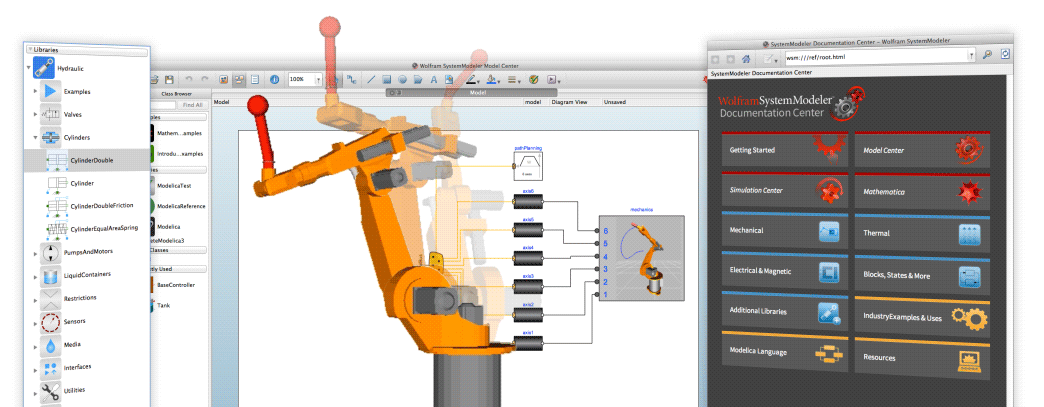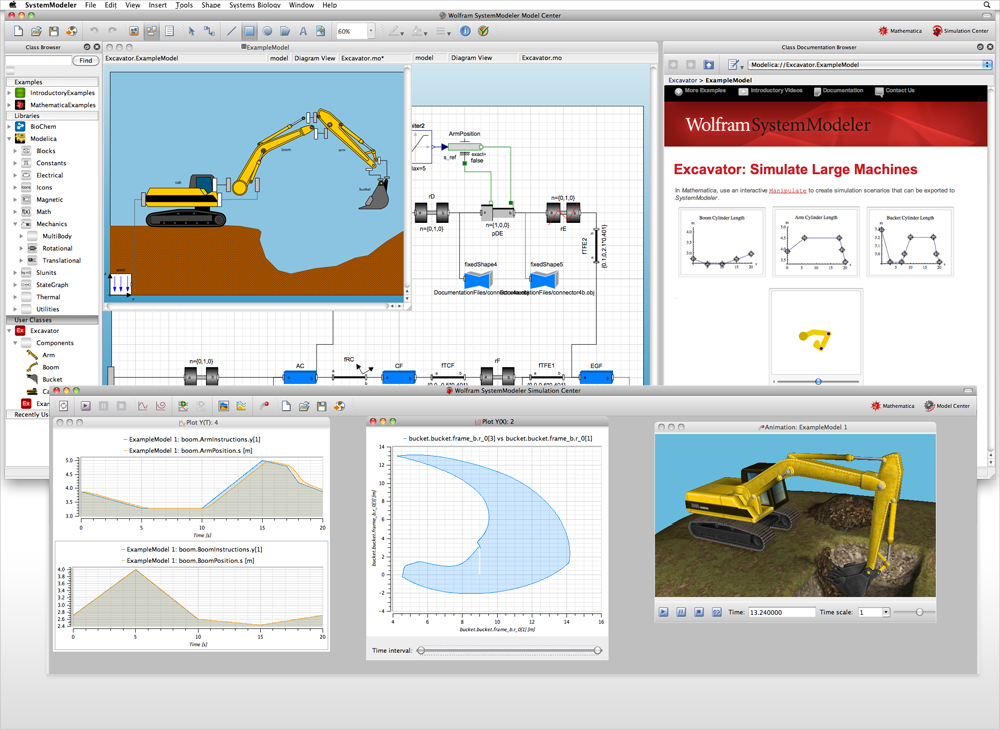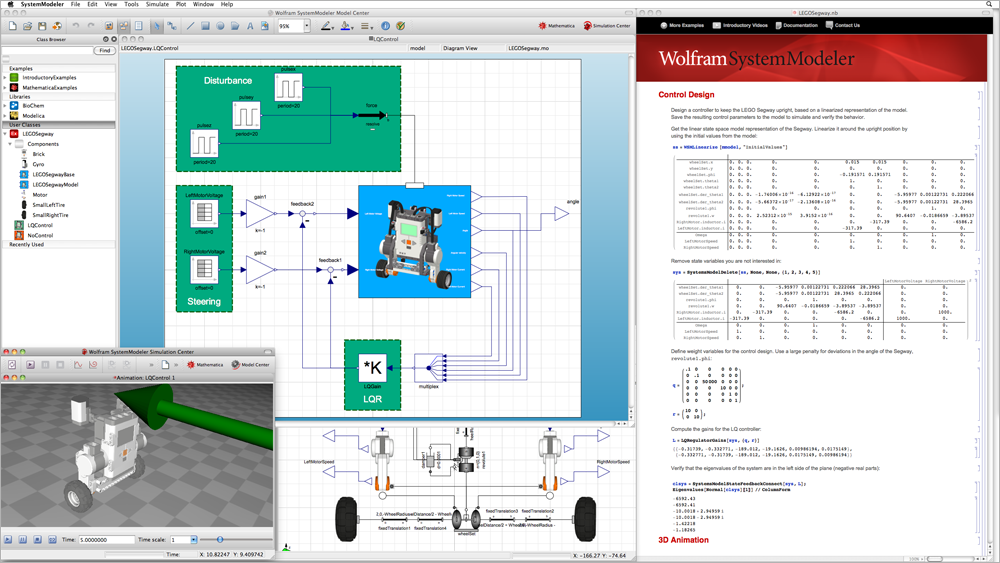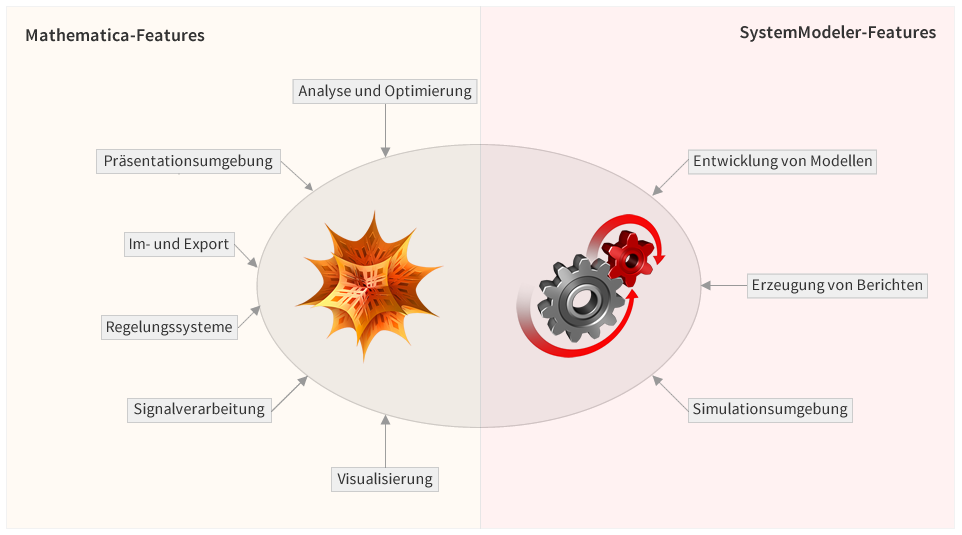# Wolfram – Wolfram SystemModeler# Wolfram SystemModeler

Wolfram SystemModeler is a software platform for simulating multidimensional, physical systems, which can be created per drag and drop thanks to the intuitive user interface.

Using the Wolfram SystemModeler, high-precision modeling simulations can be performed, because, unlike almost every other system, the SystemModeler computes not only numerically but also symbolically, i.e. with arbitrary accuracy. Numerous libraries, including the related modeling tools, simplify these multiphysical or cyber-physical simulations. The models are created by drag-and-drop components from integrated or added libraries. The liberaries contain components from a variety of technical fields, e.g. electrical, mechanical, fluidic, and chemical applications, as well as from biological applications and energy managment.

To simulate and experiment with models you typically interactively select what variables to visualize or for 3d mechanical systems automatically animate.#### Short Overview of SystemModeler's features

• Drag and drop modeling
• Hierarchical modeling
• Multidomain modeling
• Built-in model libraries
• Hybrid systems modeling
• Simulation and experimentation
• Instant visualization
• more...

## Analysis and Design with Mathematica

Bring the full power of Mathematica to your model analysis. Programmatically control SystemModeler simulations and use built-in functions for finding model equilibria, linearizing models, and more. Perform model calibration and system optimization using Mathematica's symbolic and numerical features, and present your results with advanced graphics and interactivity.

#### Short Overview of Mathematica's features

• Programmatic simulation control
• Sensitivity analysis
• Model calibration
• Notebook environment
• Plotting and custom visualization
• Model and equation analysis
• Control system design
• more...

## Licensing and Pricing

• License Type I: Wolfram SystemModeler (without Mathematica)
• License Type II: Wolfram Modeling & Simulation Suite (Wolfram SystemModeler & Wolfram Mathematica)
• Comparision between the different Types
• Pricing information for Wolfram SystemModeler can be found in our eShop.

## Videos, Tutorials, and more

A selection of videos, tutorials, and the user manual can be found here

## Wolfram Modeling & Simulation Suite SystemModeler Integration with Mathematica

The Wolfram Modeling & Simulation Suite contains SystemModeler - for modeling and simulation of models and systems – and Mathematica to programmatically control SystemModeler simulations, analyse model equations and simulation results, and present results with advanced graphics and interactivity.If you decide for Wolfram Modeling & Simulation Suite instead of Wolfram SystemModeler alone, you get these additional advantages:

• Control system design
A full suite of control systems features is built in to Mathematica, including stability and frequency analysis, visualization, and controller design. Automatically linearize SystemModeler models into Mathematica's standard state-space representation for linear time-invariant (LTI) systems. Use numerical model linearization, or preserve named parameters for further symbolic analysis.
• Sensitivity analysis
Simulate models while obtaining the sensitivity of system variables with respect to specified parameters. Sensitivity band plotting is built in. Discover which system variables are most sensitive to parameters of interest or which parameters have the greatest effect on system behavior.
• Model and equation analysis
Access model equations and properties from Mathematica and use its symbolic mathematics functions to perform analyses on system equations such as finding closed form solutions, investigating approximate solutions, or solving for optimal parameter values or special states. Automatically perform constrained or unconstrained searches for equilibrium states.
• Presentation of results
The Mathematica environment is designed to make exploration and analysis as efficient as possible. Entering commands into a Mathematica notebook automatically creates a record of your work that can be shared with colleagues or reused and improved for future analyses. Notebooks combine code, data, explanatory text, plots and graphics, and interactive elements in a single platform-independent Computable Document Format (CDF).
• Parallel Computation
The parallel computational capabilities of Mathematica, up to the docking to computing clusters, serve to reduce computing time.

© ADDITIVE GmbH. All rights, errors and amendments reserved.